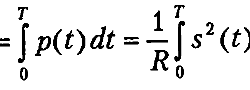## Electrical signal power and energy calculations by exampleIn electronics and signal processing, you have to deal with electrical signals. In many cases, you may need to calculate signal power and energy. Power and energy for DC In a standard situation, when DC power supply is applied to a known resistor or another device like an LED, motor you can calculate its power very easy by applying Ohms law: If we rung this device for time T then we can calculate total energy used: In some cases, you may not know the resistance of your circuit. In this case, you can measure the current flow. So your power formula can be transformed by using same Ohms law:

## Signal power and energy calculationThe most common signal characteristics are energy and power. In signal theory, these terms require additional comments because they are different from what we are using in AC or DC systems. What are power and energy? When we connect the R resistor to voltage U, the resistor will dissipate some power equal to P=U2/R. During time T, the energy loss on this resistor will be E= TU2/R. Now let us say that we add some signal s() instead of DC voltage. In this case, the power will depend on time as the signal is time-dependent. The term is called instantaneous power: p(s)=s(t)2/R